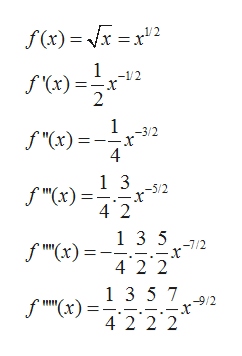# b. f(x)= x, a=1, v2

Question
1 views

Find the first 6 non-zero terms of the Taylor series expanded at the given value of x=a below and write the function that results from those terms. Then use that to estimate the given value.

check_circle

Step 1

Given function is

Step 2

Taylor series expansion formula of f(x) around point x = a is

Step 3

Now, find the deriva...help_outlineImage Transcriptionclosef(x)= x x2 1 f(x)= 2 -1/2 1 f "(x) 4 -3/2 1 3 -5/2 f "(x) 4 2 13 5 42 2 1 3 5 7 9/2 4 2 2 2 fullscreen

### Want to see the full answer?

See Solution

#### Want to see this answer and more?

Solutions are written by subject experts who are available 24/7. Questions are typically answered within 1 hour.*

See Solution
*Response times may vary by subject and question.
Tagged in

### Calculus